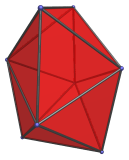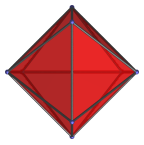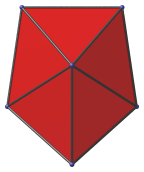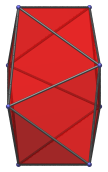# The Snub Disphenoid

The snub disphenoid is the 84th Johnson solid (J84). Its surface consists of 12 equilateral triangles, 18 edges, and 8 vertices.It is also known as the Siamese dodecahedron because it has 12 faces and can be formed by conjoining two regular octahedra each with a pair of triangles removed and the remaining 6 faces slightly deformed, like Siamese twins (conjoined twins).

Another way to construct it is to split a tetrahedron, regarded as a digonal antiprism ("disphenoid"), into two pairs of triangles, suitably deformed, and joining them with a band of 8 triangles ("snubbing"). Hence the name snub disphenoid. A similar process applied to the octahedron, regarded as a triangular antiprism, produces the regular icosahedron; and when applied to the square antiprism, produces the snub square antiprism (J85).

It is one of the special Johnson solids at the end of Norman Johnson's list that are not directly derived from the uniform polyhedra by cut-and-paste operations.

## Projections

Here are some views of the snub disphenoid from various angles:

Projection Envelope DescriptionSquare

Top view.Irregular pentagon

Side view.Irregular octagon

Oblique side view at 45° angle.

## Coordinates

The Cartesian coordinates of the snub disphenoid, centered on the origin with edge length 2, are:

• (0, √A, ±1)
• (±√C, √B, 0)
• (0, −√B, ±√C)
• (±1, −√A, 0)

where A, B, and C are the roots of the following polynomials within the indicated ranges:

 2A3 − A2 − 8A − 4 = 0, 2 ≤ A ≤ 3 2B3 + 11B2 + 4B − 1 = 0, 0 ≤ B ≤ 1 C3 − 17C2 + 64C − 64 = 0, 1 ≤ C ≤ 2

Their numerical values are approximately:

• A = 2.458190775872486
• B = 0.169022229424176
• C = 1.661955541151648

Their square roots are approximately:

• √A = 1.567861848465127
• √B = 0.411123131706519
• √C = 1.289168546448310

Last updated 18 Jun 2019.